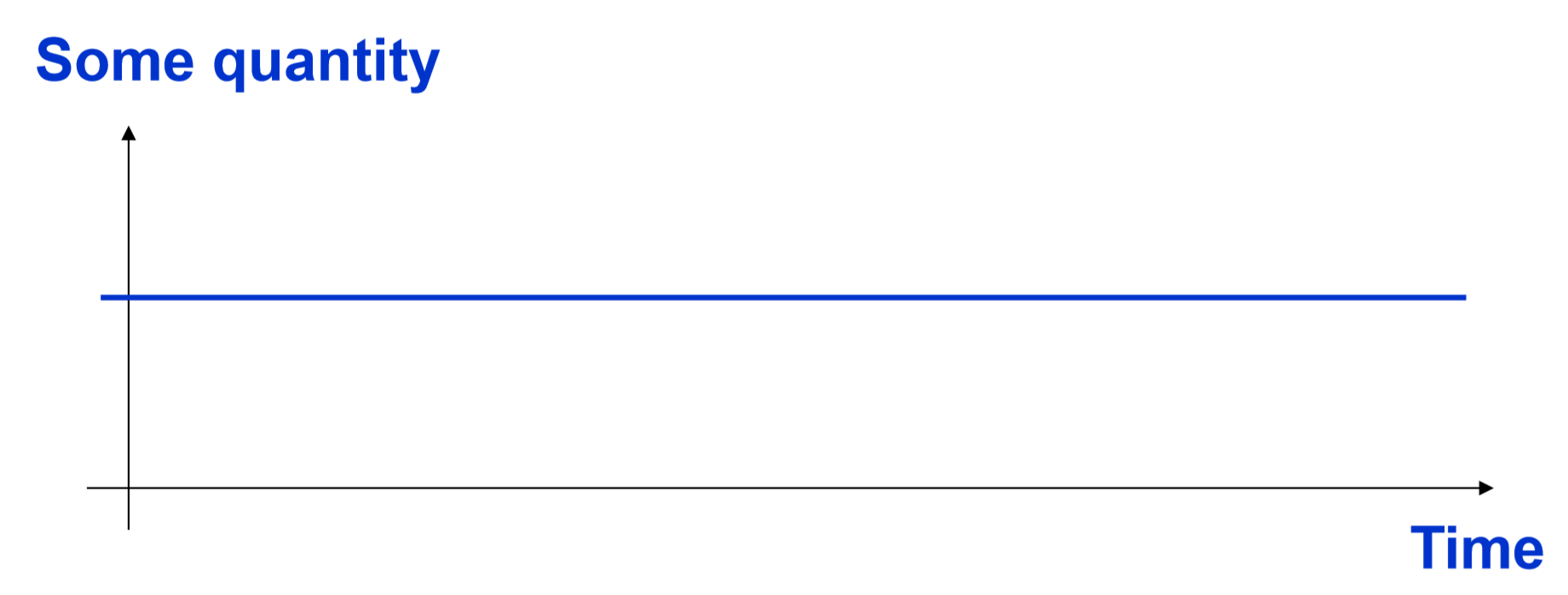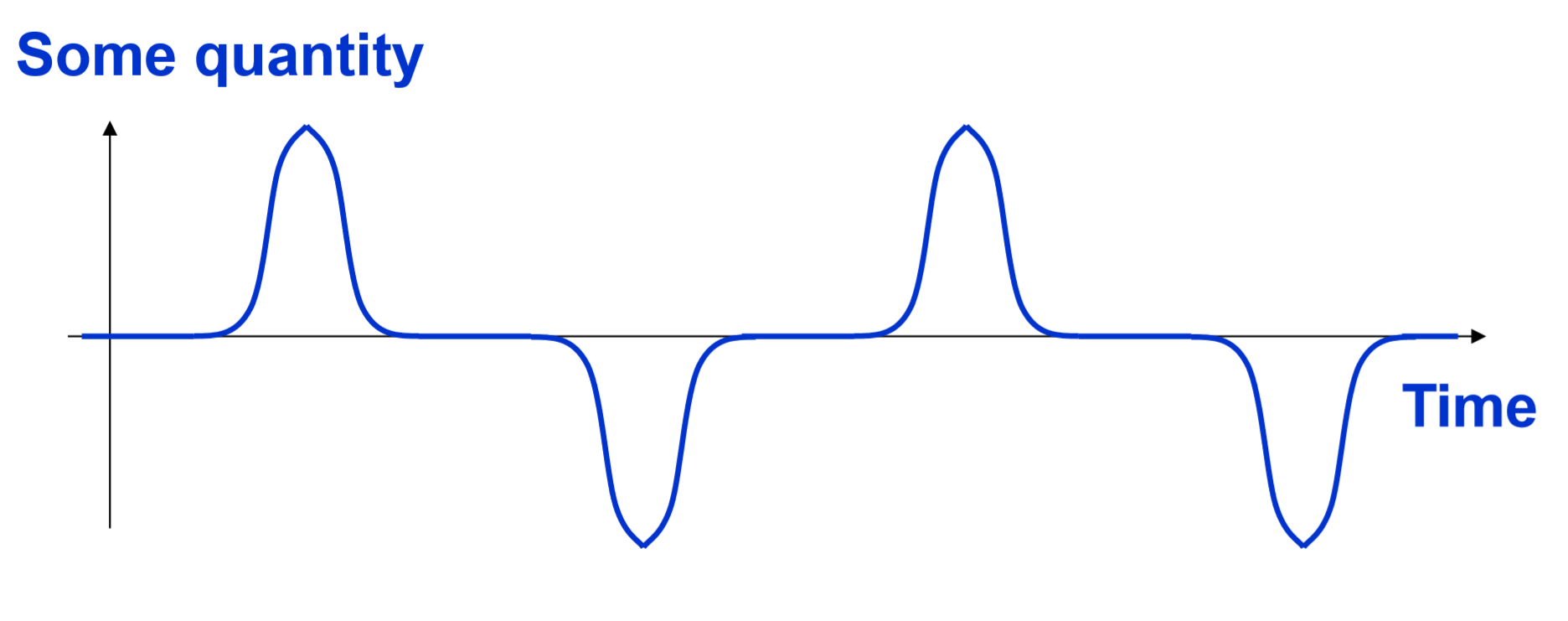Skip to main content
$$\newcommand{\id}{\mathrm{id}}$$ $$\newcommand{\Span}{\mathrm{span}}$$ $$\newcommand{\kernel}{\mathrm{null}\,}$$ $$\newcommand{\range}{\mathrm{range}\,}$$ $$\newcommand{\RealPart}{\mathrm{Re}}$$ $$\newcommand{\ImaginaryPart}{\mathrm{Im}}$$ $$\newcommand{\Argument}{\mathrm{Arg}}$$ $$\newcommand{\norm}{\| #1 \|}$$ $$\newcommand{\inner}{\langle #1, #2 \rangle}$$ $$\newcommand{\Span}{\mathrm{span}}$$

# 2.2: How to Create a Model

$$\newcommand{\vecs}{\overset { \rightharpoonup} {\mathbf{#1}} }$$

$$\newcommand{\vecd}{\overset{-\!-\!\rightharpoonup}{\vphantom{a}\smash {#1}}}$$

There are a number of approaches for scientiﬁc model building. My favorite way of classifying various kinds of modeling approaches is to put them into the following two major families:

Descriptive modeling In this approach, researchers try to specify the actual state of a system at a given time point (or at multiple time points) in a descriptive manner. Taking a picture, creating a miniature (this is literally a “model” in the usual sense of the word), and writing a biography of someone, all belong to this family of modeling effort. This can also be done using quantitative methods (e.g., equations, statistics, computational algorithms), such as regression analysis and pattern recognition. They all try to capture “what the system looks like.”

Rule-based modeling In this approach, researchers try to come up with dynamical rules that can explain the observed behavior of a system. This allows researchers to make predictions of its possible (e.g., future) states. Dynamical equations, theories, and ﬁrst principles, which describe how the system will change and evolve over time, all belong to this family of modeling effort. This is usually done using quantitative methods, but it can also be achieved at conceptual levels as well (e.g., Charles Darwin’s evolutionary theory). They all try to capture “how the system will behave.”

Both modeling approaches are equally important in science and engineering. For example, observation of planetary movement using telescopes in the early 17th century generated a lot of descriptive information about how they actually moved. This information was already a model of nature because it constituted a simpliﬁed representation of reality.

In the meantime, Newton derived the law of motion to make sense out of observational information, which was a rule-based modeling approach that allowed people to make predictions about how the planets would/could move in the future or in a hypothetical scenario. In other words, descriptive modeling is a process in which descriptions of a system are produced and accumulated, while rule-based modeling is a process in which underlying dynamical explanations are built for those descriptions. These two approaches take turns and form a single cycle of the scientiﬁc modeling effort.

In this textbook, we will focus on the latter, the rule-based modeling approach. This is because rule-based modeling plays a particularly important role in complex systems science. More speciﬁcally, developing a rule-based model at microscopic scales and studying its macroscopic behaviors through computer simulation and/or mathematical analysis is almost a necessity to understand emergence and self-organization of complex systems. We will discuss how to develop rule-based models and what the challenges are throughout this textbook.

A typical cycle of rule-based modeling effort goes through the following steps (which are similar to the cycle of scientiﬁc discoveries we discussed above):

1. Observe the system of your interest.
2. Reﬂect on the possible rules that might cause the system’s characteristics that were seen in the observation.
3. Derive predictions from those rules and compare them with reality.
4. Repeat the above steps to modify the rules until you are satisfied with the model (or you run out of time or funding).

This seems okay, and it doesn’t contain the logical problem of “proving a hypothesis” that we had before, because I loosened the termination criteria to be your satisfaction as a researcher. However, there is still one particular step that is fundamentally difficult. Which step do you think it is?

Of course, each of the four steps has its own unique challenges, but as an educator who has been teaching complex systems modeling for many years, I find that the second step (“Reﬂect on possible rules that might cause the system’s characteristics seen in the observation.”) is particularly challenging to modelers. This is because this step is so deeply interwoven with the modeler’s knowledge, experience, and everyday cognitive processes. It is based on who you are, what you know, and how you see the world—it is, ultimately, a personal thinking process, which is very difﬁcult to teach or to learn in a structured way.

Let me give you some examples to illustrate my point. The following ﬁgure shows an observation of a system over time. Can you create a mathematical model of this observation?Figure $$\PageIndex{1}$$: Observation example 1.

This one should be quite easy, because the observed data show that nothing changed over time. The description “no change” is already a valid model written in English, but if you prefer writing it in mathematical form, you may want to write it as

$x(t) = C \label{(2.1)}$

or, if you use a differential equation,

$dx /dt = 0. \label{(2.2)}$

Coming up with these models is a no brainer, because we have seen this kind of behavior many times in our daily lives. Here is another example. Can you create a mathematical model of this observation?Figure $$\PageIndex{2}$$: Observation example 2.

Now we see some changes. It seems the quantity monotonically increased over time. Then your brain must be searching your past memories for a pattern that looks like this curve you are looking at, and you may already have come up with a phrase like “exponential growth,” or more mathematically, equations like

$x(t) = ae^{bt} \label{(2.3)}$

or

$dx/dt=bx \label{(2.4)}$

This may be easy or hard, depending on how much knowledge you have about such exponential growth models. In the meantime, if you show the same curve to middle school students, they may proudly say that this must be the right half of a ﬂattened parabola that they just learned about last week. And there is nothing fundamentally wrong with that idea. It could be a right half of a parabola, indeed. We never know for sure until we see what the entire curve looks like for $$- ∞ < t < ∞$$.

Let’s move on to a more difﬁcult example. Create a mathematical model of the following observation.Figure $$\PageIndex{3}$$: Observation example 3.

It is getting harder. Unless you have seen this kind of dynamic behavior before, you will have a hard time coming up with any concise explanation of it. An engineer might say, “This is a sawtooth wave,” or a computer scientist might say,“This is time mod something.” Or, an even more brilliant answer could come from an elementary school kid, saying,“This must be months in a calendar!” (which is equivalent to what the computer scientist said, by the way). In either case, people tend to map a new observation to something they already know in their mind when they create a model.

This last example is the toughest. Create a mathematical model of the following observation.Figure $$\PageIndex{4}$$: Observation example 4.

Did you come up with any ideas? I have seen only a few people who were able to make reasonable models of this observation throughout my career. The reason why this example is so hard to model is because we don’t see this kind of behavior often in our lives. We are simply not experienced with it. We don’t have a good mental template to use to capture the essence of this pattern2.

I hope that these examples have made my point clear by now. Coming up with a model is inherently a personal process, which depends on your own knowledge, experience, and worldview. There is no single algorithm or procedure you can follow to develop a good model. The modeling process is a full-scale interaction between the external world and your whole, intellectual self. To become a good modeler, you will need to gain diverse knowledge and experience and develop rich worldviews. This is why I said it would be very difﬁcult to be taught.

Exercise $$\PageIndex{1}$$

Create a few different models for each of the examples shown above. Discuss how those models differ from each other, and what you should do to determine which model is more appropriate as an explanation of the observed behavior.

2For those who are curious—this kind of curve could be generated by raising a sine or cosine function of time to an odd number (e.g., $$sin3(t)$$, $$cos5(t))$$, but I am not sure if knowing this would ever help you in your future career.